Palago Fractals Cameron Browne 2009Visit Palagonia!

This page shows some fractal constructions (fractalagos?) and other mathematical curiosities made with Palago tiles.

Sierpinski Triangle

Figure 1 shows the initial state and first two iterations of a Sierpinski Triangle made with Palago tiles. The figures require 6, 15 and 45 tiles to complete, respectively. Thanks for Stephen Tavener for suggesting this design.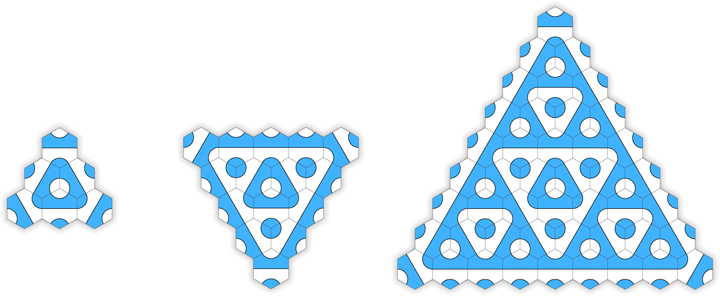Figure 1.  Initial state and first two iterations.

Figures 2 and 3 show the next two iterations of this construction.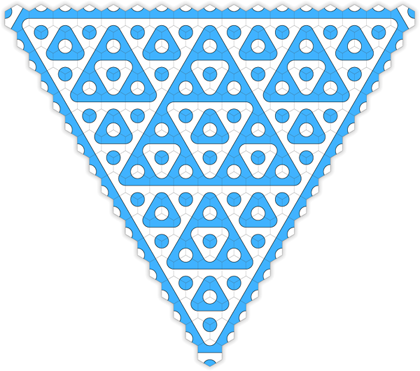Figure 2.  Third iteration (153 tiles).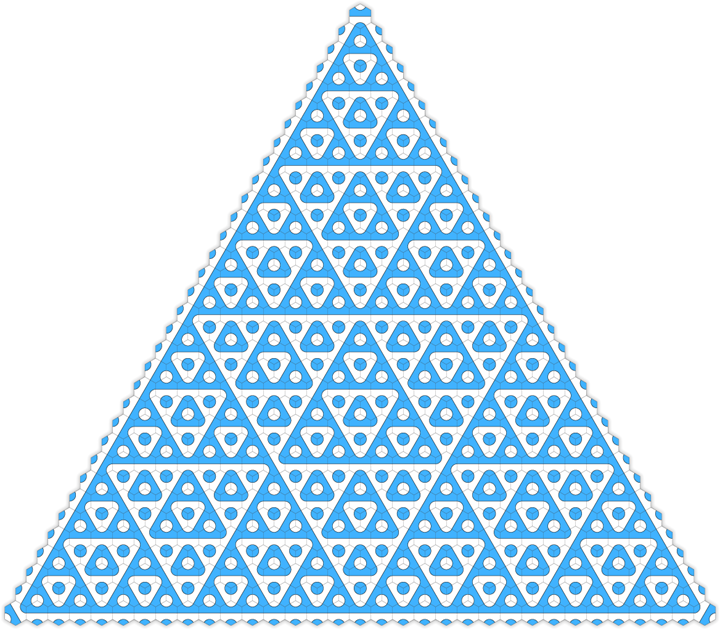Figure 3.  Fourth iteration (561 tiles).

Rose Construction

Figures 4, 5 and 6 shows the initial state and several iterations of a simple construction reminiscent of a rose in bloom.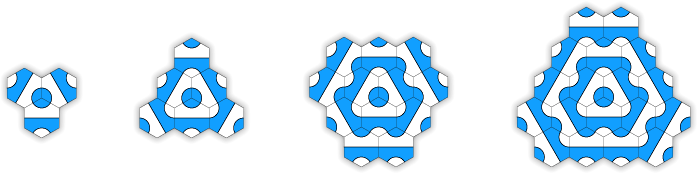Figure 4.  Initial state and first three iterations.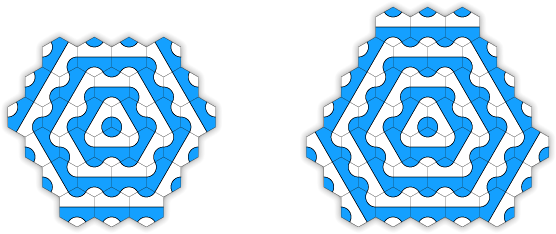Figure 5.  Fourth and fifth iterations.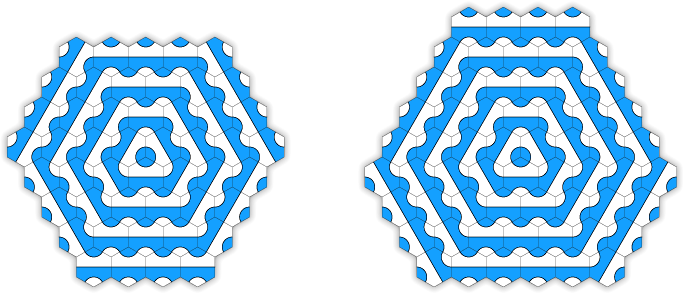Figure 6.  Sixth and seventh iterations.

Other

Figure 7 shows a variation on the Sierpinski construction that I call Day and Night as an homage to Escher.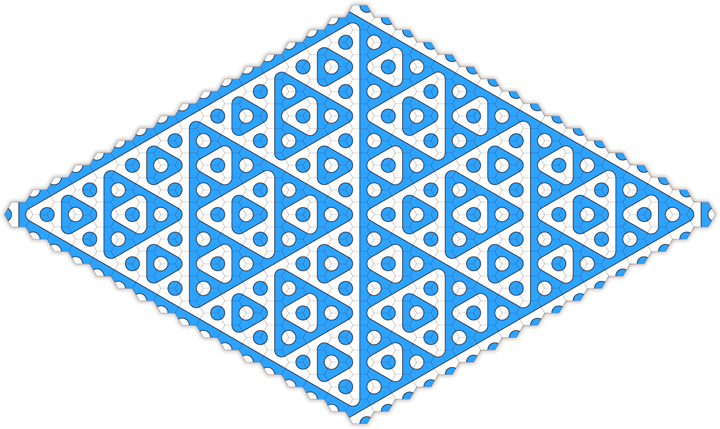Figure 7.  Day and Night.

History

Palago tiles by Cameron Browne and copyright (c) Cyberite Ltd 2008.

Home - Games

Site designed by Cameron Browne © 2009.